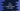# C++ program to create multiple objects of a class## C++ program to create multiple objects of a class:

In this post, we will learn how to create multiple objects of a class in C++. A class is like a blueprint. We can define the structure of the objects in the class and once we create objects, we can assign different values to these objects.

Let’s learn how to create different instances of a class in C++.

### Example program 1:

Let’s take a look at the below program:

``````#include <iostream>
#include <string>
using namespace std;

class Vehicle
{
public:
int id;
string color;
float price;
string type;
};

int main()
{
Vehicle v1, v2;

v1.id = 1;
v1.color = "red";
v1.price = 12000;
v1.type = "Type A";

v2.id = 2;
v2.color = "blue";
v2.price = 15000;
v2.type = "Type B";

cout << "Vehicle v1, id: " << v1.id << ", color: " << v1.color << ", price: " << v1.price << ", type: " << v1.type << endl;
cout << "Vehicle v2, id: " << v2.id << ", color: " << v2.color << ", price: " << v2.price << ", type: " << v2.type << endl;

return 0;
}``````

Here,

• Vehicle is a class with four public variables.
• Inside main, we are creating two objects of Vehicle, v1 and v2.
• For both of these objects, the variables are assigned different values. We can access the variables using dot notation because these are public variables.
• The last two lines are printing the values of v1 and v2.

Similarly, you can create any number of objects for the Vehicle class.

### Multiple classes using object of one in another:

Let’s try one different example with multiple classes. We will create two classes Student and Teacher. Each class can store the name, which is a string property. The Student class can also store a Teacher object, i.e. each Student object has a Teacher object. We can access the name of the Teacher object from the Student object.

Let me show you the code:

``````#include <iostream>
#include <string>
using namespace std;

class Teacher
{
public:
string name;
};

class Student
{
public:
string name;
Teacher teacher;
};

int main()
{
Student s1, s2;
Teacher t1, t2;

s1.name = "Alex";
s2.name = "Bob";

t1.name = "Charlie";
t2.name = "Daisy";

s1.teacher = t2;
s2.teacher = t1;

cout << "Teacher of " << s1.name << " is " << s1.teacher.name << endl;
cout << "Teacher of " << s2.name << " is " << s2.teacher.name << endl;

return 0;
}``````

Here,

• Teacher is the class to hold the information of a teacher. It can hold only the name of a teacher.
• Student is the class to hold the information of a student. It can hold the name of a student and another teacher object.
• We created two Student objects s1 and s2, two Teacher objects t1 and t2.
• The teacher of s1 is set as t2 and the teacher of s2 is set as t1.
• The last two lines are printing the name of each student and the name of the teacher for that student.

It will print the below output:

``````Teacher of Alex is Daisy
Teacher of Bob is Charlie``````

Similar to the above two examples, you can create any number of objects from a predefined class. If you are working in a large project, you can create one single class and use that class to create objects at different places in your project.

In the above two examples, we haven’t used any methods in the classes, but you can also create different methods to read or edit the properties of an object. For example, you can create a method to set the name of the objects and instead of accessing the name properties in the objects directly, this method can be called.

The second example shows how to keep objects inside another class objects. We can keep different types of objects in a class object and access the properties of these objects.